# Computational Investigation on Single Cylinder Spark Ignition Engine Using Gasoline-propane in Dual Fuel Mode

Computational Investigation on Single Cylinder Spark Ignition Engine Using Gasoline-propane in Dual Fuel Mode
National Institute of Technology, Hazratbal Srinagar 190006, India

Faculty of Engineering, Mechanical Engineering Department, NIT Srinagar 190006, Kashmir, India

Corresponding Author Email:
Page:
457-465
|
DOI:
https://doi.org/10.18280/ijht.370211
15 February 2018
|
Accepted:
14 May 2019
|
Published:
30 June 2019
| Citation

OPEN ACCESS

Abstract:

This study focused on the computational and parametric research on a single cylinder spark ignition engine using dual-fuel, 100 % gasoline and (10 %, 20 %, 30 %) propane in gasoline on volume basis as an alternative fuel for investigating the performance and emission characteristics of spark ignition engine. The performance parameters Brake power, Torque, Bsfc, and Bmep were examined using Avl Boost software. In addition, the exhaust emissions like nitrogen oxide, carbon monoxide, hydrocarbons were also measured. The (10 %, 20 %, &30 %) of propane in gasoline decreases in power compared to 100 % gasoline, however engine fuelled with 10 %, 20 %, and 30 % propane in gasoline shows reduction in specific fuel consumption. The study investigates the effect of dual-fuel propane and gasoline on a four-stroke single cylinder spark ignition engine. The study was carried out at varying percentage load and varying compression ratio for constant engine speed. The focus of this parametric study tries to investigate the effects of replacing individual gasoline and propane with the optimum mixture of 10 %, 20 %, and 30 % propane in gasoline on volume basis in spark ignition engine in order to improve its performance and emission characteristics.

Keywords:

performance, emissions, engine, simulation, alternative fuel

1. Introduction

Petroleum is a conventional fuel used in internal combustion engines and involves expensive and high-end technology for its exploration and production. The most common alternative fuels are CH4 (Methane), C3H8 (Propane), CH3OH (Methanol), CH3CH2OH (Ethanol), and H2 (Hydrogen). Researchers have done a lot of work on alternative fuels and its applications on internal combustion engines. Liquefied petroleum gas (propane) has widely been adopted as an automotive fuel because of its potential for reducing emissions, relatively low fuel price, and improved performance . Propane which is the main constituent of liquefied petroleum gas is determined very neat alternative fuel for the engine. Propane has good combustion properties and can be stored safely. Propane has less number of carbon atoms than conventional gasoline fuel and hence it produces less CO emissions during combustion proving it to be a cleaner alternative fuel. And when propane fuel is used in spark ignition engine it improves its performance and reduces the emissions. Propane is a useful alternative fuel used in commercial buses and loaded trucks which are running in those areas that are prone to air pollution. Liquefied petroleum gas mainly consists of propane, with a percentage (~40 %) of butane . Currently, propane fuel is used in cars mostly in developed countries (like USA, Japan, Netherlands, France, Italy). But very few researchers have used these fuels together either in blended form or individually in a dual-fuel mode in the same engine [3-4]. Ethyl Mercaptan is a powerful odorant which is used for detecting the leak in the container containing liquefied petroleum gas. Nitrogen oxide is another greenhouse gas with adverse effects on the global environment . The NOx emissions are known to be directly related with air-fuel ratio and temperature inside the combustion chamber .

In the struggle to decrease emissions and to make fuel more economical the liquid injection of fuel is preferred over vaporization of gas and the open loop control system is being replaced with the closed loop which has been tested in many engines. Engines fuelled with propane are highly in demand, have a mixer adjustment that provides fuel in gas form and send it to the intake manifold where it mixes with the air , unlike the traditional mixers which face the difficulty in maintaining the A/F ratio and do not meet the standards of emission regulations . Another advancement to the mixer adjustment is the gaseous fuel injection system where the propane gas port injection is used resulting in reduced problems of injection systems and the Air/fuel ratio control in both light and heavy duty vehicles .

An important characteristic of propane is its higher octane number than gasoline which permits higher compression ratio resulting in higher thermal efficiency [4, 10]. Engines running on propane operate smoothly due to gaseous nature of the fuel. Fuel consumption using propane is also less than gasoline due to higher energy content of propane. While combustion of propane produces very few emissions compared to gasoline, it shows a slight reduction in power because of poor volumetric efficiencies . However, this reduction in power can be improved by several alternative methods provided by various researchers. One of the methods is to increase the compression ratio which results in improving the performance of engine under naturally aspirated conditions and by applying supercharger or turbocharger, which helps in supplying more air to the engine for the combustion of fuel [4, 10-12]. Gaseous Light-Duty vehicle cannot attain this advantage and hence do not have optimal engine efficiency [7, 13]. Variable compression ratio (VCR) technology has long been recognized as a method for improving the fuel economy of SI engines. From the last many decades, various engine designs using variable compression ratio have been given. A lot of work needs to be done in this field to maximise the fuel efficiency and to make it commercially feasible for the practical applications. For example, when the engine operates at lower speeds, the intake capacity of air is also less and the compression ratio needs to be increased in order to increase the power of the engine . Most of the previously published work is the comparison of different fuels under varying speed in spark ignition engines. But limited number of publications is on spark ignition engine which have constant speed, working on variable percentage load and varying compression ratio. Bayraktar and Durgun  have developed an engine working on LPG and gasoline in dual-fuel mode, and compared their performance and emission characteristics. Commercial fuels contain blends of hydrocarbon series of classes, alkanes (paraffin’s), cycloalkanes (naphthenes), alkenes (olefins) and aromatics. A practical measure of fuel’s resistance to knock is vital and is determined by the octane number of the fuel, a higher octane number indicates more resistance to knock .

In this work, we have used a small scale single cylinder spark ignition engine for checking its performance and emission characteristics fuelled with propane and gasoline in dual-fuel mode. The Avl Boost software was used as the simulation tool for one particular engine model Industrial plus with a 6.5 horsepower air-cooled single cylinder four stroke spark ignition engine, with external carburetion from Briggs & Stratton. We have controlled the power losses obtained for pure propane by using dual-fuel (propane and gasoline), though the power output for the pure propane shows decrease compared to gasoline, but the lower emissions make this fuel a strong contender for commercial applications. Moreover, a comparison has been made between two fuels, gasoline (100 %) and percentage of propane (10 %, 20 %, and 30 %) in gasoline on volume basis for checking its performance and emission characteristics, under varying engine operating conditions. An engine model was developed in Avl Boost for predicting the performance and exhaust emissions of single cylinder SI engine. Since each fuel has its own combustion characteristics and properties. So each fuel was used separately as 100 % gasoline, and percentage of propane i.e. (10 %, 20 %, and 30 %) in gasoline as dual-fuel. The pure gasoline fuel shows more emissions compared to 10 %, 20 %, and 30 % propane in gasoline on volume basis. In this contribution, we have used vibe 2-zone combustion model for considering both burned and unburned zone of the combustion chamber  and finally for checking heat transfer in engine, the woschni heat transfer model, was used. The percentage of propane in gasoline fuel, i.e. 10 %, 20 %, and 30 %, by volume, and the results obtained both performance and emission characteristics were compared with 100 % gasoline fuel. While comparing 100 % pure gasoline with 10 %, 20 %, & 30 % of propane in gasoline on volume basis the performance improved for all the percentage of propane i.e. 10 %, 20 %, & 30 % in gasoline fuel with varying compression ratio, and emissions decreased compared to 100 % gasoline. The same percentage of propane fuel in gasoline at varying percentage load shows less power as compared to 100 % gasoline. Exhaust emissions like NOx, and CO decreased for 100 % gasoline for varying percentage load.

2. Simulation Setup

## 1.png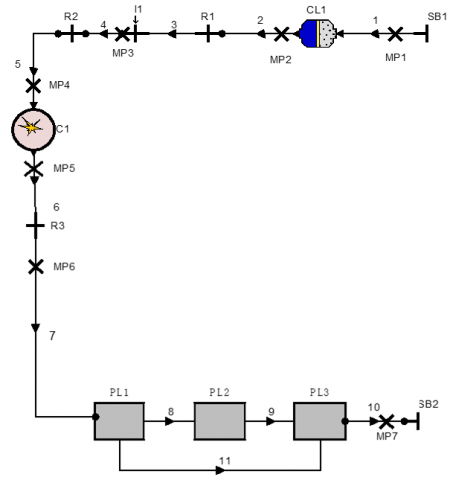Figure 1. Layout of gasoline engine model

The one-Dimensional Simulation model of engine was designed for predicting the engine performance and exhaust emissions working on gasoline and propane in dual-fuel mode, under varying compression ratio and varying percentage load. The simulation software model was used for performing the simulations on a single cylinder spark ignition engine. This Engine model has been graded by Avl Boost and its layout is shown in Figure 1, further specifications of the engine are shown in Table 1. The various elements of Avl Boost Software enable the user to develop a model of one- Dimensional engine test bench setup by using the pre-defined elements which are present in the software toolbox. All these elements are joined by various connectors for making the engine model complete by using pipelines . In Figure 1, the engine is denoted by E1, and C1 represents the single cylinder SI Engine.Mp1 to Mp7 denote measuring points. Pl1 to Pl3 denote plenum. System boundary is shown by SB1 and SB2, and the flow pipes are from 1 to 10.CL1 represents the cleaner, R1 to R3 are restrictions .

3. Model Foundation

The foundation equation used for the formulation of model is basic thermodynamic equation which is obtained from the first law of thermodynamics:

$\partial \mathrm{E}=-\partial Q-\partial W+\sum_{i} h_{i} d m_{i}$  (1)

where E is the internal energy, the exchange of heat between gas and wall of the cylinder. W is work done,hi is the specific enthalpy,dmi is flow of mass inside or outside of the cylinder,dw can be denoted as p dv, where p is pressure and v is volume of the cylinder .

3.1 Mathematical model

Mathematical models for spark–ignition engines can be divided into two main groups’ dimensional and thermodynamic models. Thermodynamic models can, in turn, be classified in two sub groups single zone and multi-zone models, where as dimensional models can be divided into one and multi-dimensional models .

The charge of the cylinder is assumed to be uniform in temperature, pressure, and composition for single zone models. The function of these models is used as predictive tools or diagnostic (heat release analysis). Because of the simplicity of single zone models; it is used for calculating the flows into and out of crevices. However single zone models did not consider the combustion chamber geometry and flame propagation. Single zone models can also be used as predictive tools if the mass burning rate is specified. On combustion mass duration, burning rate depends, ignition angle, residual mass, engine geometry, equivalence ratio, etc. Therefore tuning may be required for predicting the diagrams of pressure in the same engine or different engine operating under variable conditions.

Multi-zone models are more feasible for resolving the combustion phenomena, compared to single zone models in a more physical manner. The chamber for combustion is generally divided into burnt and unburnt regions and sometimes thermal boundary layers in burnt and unburnt gases are also considered. The cylinder charge is normally composed of ideal gases and the first law of thermodynamics, state equation, mass conservation and volume are applied to the burnt and unburnt gases .

3.2 Single – Zone models

In single zone models the pressure, temperature, and composition of the cylinder charge are assumed to be uniform. Multi-zone models permit a more accurate treatment of the thermodynamic properties of the cylinder mixture; the burnt and unburnt gases are considered as independent thermodynamic systems that are uniform in state and composition. However, the geometry of each zone must be tracked in order to calculate the heat transfer and composition of the burnt and unburnt gases and are considered as independent thermodynamic systems that are uniform in state and composition. In single zone models, combustion can be considered as a heat addition process, and the chamber charge is regarded as a simple fluid.

The first law of thermodynamics for open system can be written as

\begin{aligned} \frac{d_{(m e)}}{d_{\theta}} &=-p \frac{d V}{d \theta}-\frac{d Q}{d \theta}+\sum{\dot{m}i} h i \\ e &=e^{0}+\int_{T_{0}}^{T} C_{v} d T \end{aligned}  (2)

where m, T, and p are the mass, temperature, and pressure, of the cylinder charge, respectively, e is the mixture specific internal energy, Cv is considered as specific heat at volume constant, V is the combustion chamber volume; dQ/dθ represents the heat losses; specific enthalpy of gases is hi, flowing into the cylinder with a mass flow rate equal to ṁi,T0 is a reference temperature e0 is the internal energy of formation at the reference temperature T0, and θ is the crank shaft angle. In the absence of injection and flows into crevices, dm/dθ = 0. In premixed charge engines, there are flows into and out of crevices (i.e. volumes between the piston, cylinder wall, and piston rings and the spark plug threads). The crevices can be modelled as a single volume at the cylinder pressure or as a series of volumes, connected by restrictions to simulate the piston ring- cylinder wall region and blow by.

Equation (1) can be written as

$\frac{d Q_{C H}}{d \theta}=m C_{v} \frac{d T}{d \theta}+p \frac{d V}{d \theta}+\frac{d Q}{d \theta}+(h-e) \frac{d m_{C R}}{d \theta}$  (3)

where dQCH /dθ represent the heat released by combustion, dmCR/dθ represents the mass flow rate into crevices, and h is the specific enthalpy.

Conservation of mass applied to the combustion chamber yields

$\frac{d m}{d \theta}=-\frac{d m_{C R}}{d \theta}$   (4)

When the cylinder pressure is high $\frac{d m_{C R}}{d \theta}>0$ and the value of h corresponds to that in the combustion chamber:

h = e + PV/m   (5)

However, during the expansion stroke, dmCR/dθ< 0 and the value of h is that of the gases contained in the crevices.

If the crevice volume and temperature are assumed constant and the crevice pressure is equal to that of the cylinder charge, the mass flow rate into the crevices can be written as

$\frac{d m_{C R}}{d \theta}=V_{C R} \frac{d p / d \theta}{R T w}$  (6)

where the crevice temperature was set equal to the temperature of the cylinder wall Tw and VCR is the crevice volume. Equation (5) can be substituted into equation (2) and (3) to obtain an equation for the heat released by combustion once the heat transfer losses dQ/dθ are specified .

Here is the crank angle θ.

4. Heat Transfer Model

The Woschni model  for the high-pressure cycle is outlined as follows.

$\propto=130 . D^{-0.2} \cdot p_{c}^{0.8} \cdot T_{c}^{-0.53} \cdot\left[C_{1} \cdot c_{m}+C_{2} \frac{V_{D . T_{C, 1}}}{P_{C, 1 . V_{C, 1}}} \cdot\left(p_{c-p_{c, 0}}\right)\right] 0.8$  (7)

C1 = 2.28+ 0.308. Cu/Cm

C2 = 0.00324 Direct Injection

D        Bore of cylinder

cm      piston mean speed

Cu   velocity circumferential

VD    cylinder displacement

Pc, o engine cylinder pressure [bar]

Tc,cylinder temperature at intake valve closing (IVC)

Pc, 1  cylinder pressure IVC [bar]

This heat transfer model which is modified form of woschni model has been published in 1990 (C6) for the purpose to give prediction of heat transfer more accurate at part load operation .

$\propto_{w}=130 . D^{-0.2} \cdot p_{c}^{0.8} \cdot T_{c}^{-0.53} \cdot\left\{c_{1} \cdot c_{m} \cdot\left[1+2\left(\frac{V_{T D C}}{V}\right)^{2} \cdot \operatorname{Imep}^{-0.2}\right]\right\}^{0.8}$   (8)

VTDC     cylinder TDC volume

V        cylinder volume actual

IMEP   is indicated mean effective pressure

In the case that

$\mathrm{C}_{2} \frac{V_{D} \cdot T_{c, 1}}{p_{c, 1} \cdot V} \cdot\left(p_{c}-p_{c, 0}\right) \geq 2 . C_{1} \cdot c_{m}\left(V_{T D C / V}\right)^{2} . \operatorname{Imep}^{-0.2}$

In this equation the calculation of heat transfer coefficient published in 1978 for the process of exchanging gas, woschni use the above equation for the heat transfer coefficient .

$\propto=130 . D^{-0.2} \cdot p_{c}^{0.8} \cdot T_{c}^{-0.53} \cdot\left(C_{3} \cdot c_{m}\right)^{0.8}$   (9)

C3 = 6.18+0.417.cu/cm

αw      is heat transfer coefficient

D      Bore of cylinder

Cm     piston mean speed

Cu     circumferential velocity

5. Combustion Models

The heat released by the burning of the mass fraction is described by a vibe function. However assuming burned charge has equal temperature is discarded. Alternately for both burned and unburned charge the first law of thermodynamics is applied respectively .

$d m_{b} u_{b} / d_{\propto}=-p_{c} \frac{d \mathrm{v}_{b}}{d_{\propto}}+\frac{d Q_{F}}{d_{\propto}}-\sum \frac{d Q_{w b}}{d_{\propto}}+h_{u} \frac{d_{m b}}{d_{\propto}}-h_{B B, b} \frac{d m_{B B, b}}{d_{\propto}}$  (10)

$d m_{u} u_{u} / d_{\propto}=-p_{c} \frac{d_{V u}}{d_{\propto}}-\sum \frac{d Q_{w u}}{d_{\propto}}-\sum \frac{d Q_{w u}}{d_{\propto}}-h_{u} \frac{d_{m b}}{d_{\propto}}-h_{B B, u} \frac{d m_{B B, b}}{d_{\propto}}$  (11)

Index b indicates burned zone and index u indicates unburned zone.

The term $h_{u} \frac{d m_{B}}{d_{\propto}}$ defines the flow of enthalpy from unburned to the burned zone converting the fresh charge to products of combustion. And heat flux is neglected between the two zones .

All volume change must result in the change of cylinder volume and the sum of the volume of the zone must be equal to the volume of the cylinder.

$\frac{d V_{b}}{d_{\propto}}+\frac{d V_{u}}{d_{\propto}}=\frac{d V}{d_{\propto}}$  (12)

Vb + Vu = V  (13)

The mixture burnt is obtained from the vibe function at each time step that is mentioned by the user. For other terms like heat losses of wall etc. models which are similar to the models that are single zone with approximate distribution on models that are two zones are used .

In spark ignition engine the performance and exhaust emissions are affected directly by the type of fuel used. These include Power, Torque, Bsfc, and Bmep, as performance parameters and exhaust emissions like Nitrogen oxide, HC emissions, and carbon monoxide. Both performance and exhaust emissions were calculated independently for varying values of percentage load, and compression ratio on single cylinder SI engine, whose specifications are mentioned in Table1.

6. Results and Discussion

Since Spark ignition engine is made to run on gasoline, the power obtained is more when running on the conventional gasoline engine. All the alternative fuels have octane number more than that of conventional gasoline fuel so will have a higher compression ratio and when the engine is operated with these fuels the performance of engine is improved.

## 2.png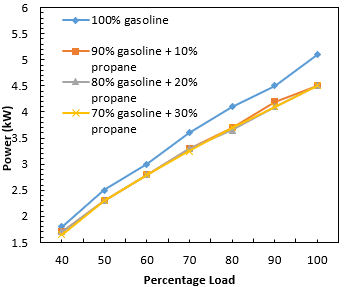Figure 2. The plot between power and percentage load for gasoline & various blends of gasoline -propane

## 3.png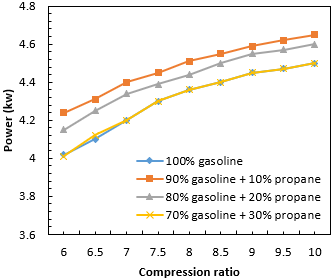Figure 3. The plot between power and compression ratio for gasoline & under various blends of gasoline-propane

Figure 2 shows the plot of power produced from various blends of gasoline and propane by varying the percentage load. It is clearly defined that the maximum power is obtained for 100 % gasoline. After increasing the percentage of propane i.e. 10 %, 20 %, 30 % in gasoline fuel, the power decreases with increase in load. The dual-fuel (propane in gasoline), however, has the same power variation for different percentage loading irrespective of the amount of propane present in the gasoline. Power loss in propane blends can be attributed to the low flame speed and standard ignition timing of propane. At compression stroke, the presence of propane in the air-fuel mixtures, when it passes through the piston ring gap reduces the air-fuel mixture quantity for required optimum combustion.

A comparison of power produced for different values of compression ratio keeping the percentage load constant is shown in Figure 3. The increase in power with the increase in compression ratio shows an increase in thermal efficiency of the engine. At higher compression ratio power obtained during combustion process is higher due to high pressure. It is accepted that oxygen content and heat of vaporization of alternative fuels are higher than gasoline fuel. This increases brake thermal efficiency and volumetric efficiency using alternative fuels. It can be clearly seen that the composition 90 % gasoline & 10 % Propane is most efficient in terms of performance. As the power shows Increase than other propane gasoline duel fuel mixtures. The possible reason for this increase in power is that by addition of propane increases its heating value which results in more power. More over propane has higher octane no. which results in more power at higher compression ratios.

## 4.pngFigure 4. The plot between torque & percentage load for gasoline & various blends of gasoline- propane

As observed in Figure 4 maximum torque is obtained at 100 % load using gasoline as the fuel. In general, the maximum torque for pure gasoline is more compared to propane fuel. The best value obtained for torque with gasoline is 14 Nm; it is also observed that torque obtained for propane is less than that of the gasoline fuel.

In Figure 5, increase in compression ratio produces higher torque which results in greater push on the piston. It is clearly shown after increasing compression ratio, torque also increases for dual-fuel at all percentages of propane i.e. (10 %, 20 %, and 30 %). The torque is almost more for every blend, but it is more for 90 % gasoline and 10 % propane. Since increase in compression ratio increases the torque because propane has more octane number than gasoline and can be used at higher compression ratios which increases the torque for all the propane blends in gasoline and proves to be efficient.

## 5.png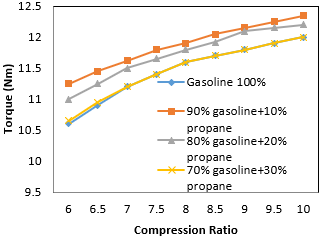Figure 5. The plot between torque & compression ratio for gasoline & various blends of gasoline -propane

## 6.pngFigure 6. The plot between BMEP & varying percentage load for gasoline and various blends of gasoline-propane

Figure 6 represents the comparison of various fuels for predicting the Bmep of the engine. For naturally aspirated engine the maximum range of Bmep are 7.5-8.5 bar at the load where torque obtained is maximum. At the percentage load where power produced is maximum the values of Bmep are 10-15 % lesser. There is variation in brake power and Bmep basically due to variation in volumetric efficiency . In Figure 6, the Bmep shape and trend follows the curve of volumetric efficiency. There is the reduction in Bmep when using propane after the load range is increased beyond 50%. Some of the loss in Bmep is due to lower flame speed of propane and longer ignition delay.

## 7.png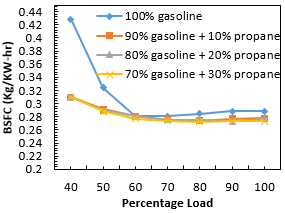Figure 7. The plot between BSFC and percentage load for gasoline & various blends of gasoline-propane

Bsfc graph at varying load is shown in Figure 7. Bsfc shows decrease with increase in load on the engine. Bsfc decreases for all the percentage of propane in gasoline after increasing load. The reason behind this decrease of Bsfc is the higher mass heating value of the fuel. Bsfc was typically increased for 100 % gasoline at lower loads. With the use of the mixture containing 10 %, 20 % and 30 % propane, Bsfc was observed to be decreased in all the cases. With the use of 100 % gasoline, Bsfc increased with a decrease in load. From the Bsfc graph, engine fuelled with propane percentage in gasoline is more efficient in terms of fuel consumption compared to gasoline fuel individually. This is because of the different properties of two fuels, where propane has the higher stoichiometric air-fuel ratio and has more heating value as compared to gasoline, so more specified amount of heat is released using less amount of fuel .

## 8.png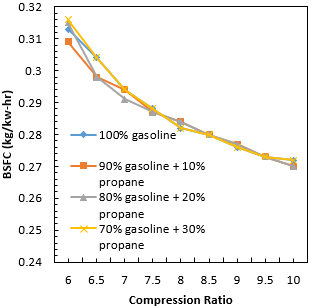Figure 8. The plot between brake specific fuel consumption (BSFC) & compression ratio for gasoline & various blends of gasoline-propane

In Figure 8, Bsfc decreased with increase in compression ratio. This is due to the reason that propane has less brake specific fuel consumption than gasoline. Propane also has the higher stoichiometric air-fuel ratio and has higher heating value compared to gasoline, so the specified amount of heat released is high with less amount of fuel. With increase in compression ratio almost all the fuels show decrease in Bsfc. This shows that when compression ratio increases the fuel proves to be efficient. Since propane has more octane number than gasoline so it can be used at higher compression ratios and consumption of fuel also decreases with increase in compression ratio.

## 9.png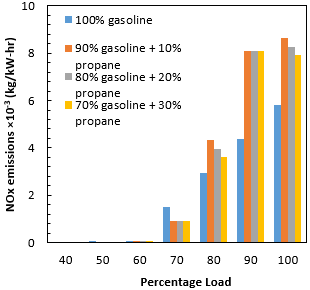Figure 9. The plot between NOx and percentage load for gasoline & various blends of gasoline –propane

## 10.png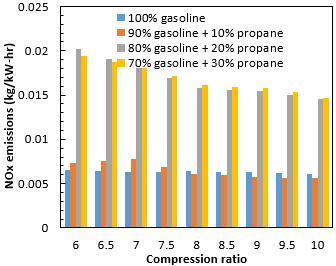Figure 10. The plot between NOx and compression ratio for gasoline & various blends of gasoline –propane

Figure 9 shows the NOx emissions with respect to the percentage load. NOxemissions show slight rise with the increase in load for 100 % gasoline as mentioned in the plot, enlarging the lean combustion region. The NOx emission increased with increase in pressure and temperature by changing the compression ratio, because the NOx emission is mostly affected by the ignition ratio and excess air ratio. The NOx is mostly increased for propane with the increase in load for all percentage of propane blends in gasoline i.e. (10 %, 20 %, &30 % propane). As the percentage load increases NOx emissions shows increasing trend, since air goes inside the combustion chamber is less which results in incomplete combustion and higher temperature inside the combustion chamber increases the NOx emissions. The higher NOx emission at increasing load can be improved by retarding the ignition timing or by using lean combustion region.

In Figure 10 the concentration of NOx is reduced at the richer side because of lower oxygen content due to combustion reactions. With an increase in temperatures, the shift in dissociation equilibrium is the reason for low NOx concentrations, also in the expansion stroke, the dissociation reactions freeze. In rich burn engines, lowering the air/fuel ratio limits the availability of O2 inside the cylinder .

## 11.png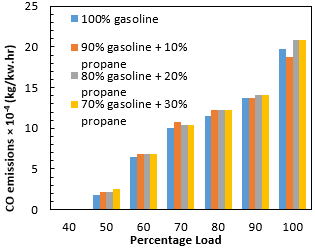Figure 11. The plot between CO and percentage load for gasoline & various blends of gasoline- propane

Figure 11 shows the CO emission with respect to the percentage load. The CO emission formation with gasoline is higher compared to propane. The formation of CO emission with a compression ratio of 9 increased after increasing load. Carbon monoxide is highest for 10 % propane and 90 % gasoline used in dual-fuel mode. This rise in CO emission is due to the insufficient time for oxidizing CO into CO2 because of getting shorter combustion duration under high temperature and pressure.

## 12.pngFigure 12. The plot between CO and compression ratio for gasoline & various blends of gasoline –propane

Propane contains less number of carbon atoms than gasoline, so emission formation is less compared to gasoline. Propane powered vehicles produce 50 percent less carbon monoxide emissions per kilometre. Therefore exhaust emissions are reduced more by the use of propane. While increasing the compression ratio the CO level decreases as shown in Figure 12 which defines that there is complete combustion after increasing compression ratios . The dual fuel composition 90% gasoline and 10 % propane shows more CO emissions with increase in compression ratio. This is due to the reason that mixture is rich as the fuel goes more in the combustion chamber and the supply of air decreases results in more CO emissions. As we start increasing the composition of propane the CO emissions also decrease because of gaseous nature of LPG which burns smoothly and complete combustion takes place.

## 13.png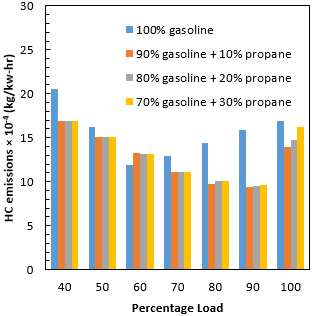Figure 13. The plot between HC and percentage load for gasoline & various blends of-gasoline- propane

Exhaust hydrocarbon measurements (HC) are presented in Figure 13. The hydrocarbon emissions increased at lower loads, however, the hydro-carbon emissions for dual-fuel stayed very low, less than 0.001 kg/kW-hr, at higher loads. But same is not the case with 100 % gasoline. It can be seen from the graph that hydrocarbon emissions are higher for gasoline compared to propane and gasoline in blended form. After increasing the percentage load hydrocarbon emission decreases and then again increases above 90 % loading. This is due to the reason, some fuel molecules in the engine do not burn or burn partially at higher loads.

In Figure 14, hydrocarbon emission shows variation for each blend. The Geometry of combustion chamber and engine operating parameters influence the hydrocarbon component spectrum . The source of Hydro carbon in engines is bulk quenching of the flame in that fraction of the engine cycles where combustion is especially slow. And some fuel remains unburned due to fuel going in crevices where there is less supply of air enters and results in formation of hydrocarbons. Such conditions are most likely to occur during transient engine operation when the air-fuel ratio, spark timing, and the fraction of the exhaust recycled for emission control may not be properly matched.

## 14.png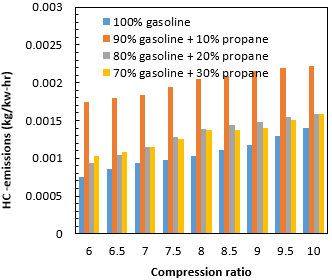Figure 14. The plot between HC and compression ratio for gasoline & various blends of gasoline –propane

7. Conclusions

In the present study, the power losses obtained from pure propane has been controlled. Brake power, Torque, Bsfc, and Exhaust emissions for varying propane percentages on a single cylinder SI engine were investigated. Simulations were done under varying load and at constant engine speed. The variation in power, torque, Bsfc, and emissions was examined. Results obtained in this investigation are mentioned below

• The brake power shows the continuous decrease with 30 % propane usage. With 10 %, & 20 %, propane usage power decreases with varying percentage load. Bsfc decreases with the increase in propane usage level and the minimum Bsfc value was obtained at 30 % propane usage.
• The engine performance shows improvement only at 10 % propane mixture ratio. When using 10 % propane as dual-fuel the Bsfc shows decreasing trend and Bmep remained the same.
•  The exhaust emissions showed positive results only for hydrocarbons. The exhaust emissions for NOx obtained are less at 100 % gasoline. But for HC and CO emissions were increased at 100 % gasoline usage.

Simulations were also performed at constant engine speed at variable compression ratios. The variations in brake power, torque, Bsfc, and emissions were predicted. Results for investigation are summarized below.

• A variable compression ratio spark ignition engine was made as the global parameter in Avl boost software and the compression ratio was varied from 6 to 12 for SI engine.
• The simulation was conducted using two fuels namely gasoline 100 % and percentage of propane i.e. 10 %, 20 %, & 30 % in gasoline as dual-fuel. The predicted performance and emission characteristics were examined.
• The power increases at varying percentage of propane. The brake power increased at 30 % usage of propane in gasoline as dual-fuel. For 10 %, 20 % propane usage, power increased after varying percentage load. The decrease in Bsfc using propane and the value of minimum Bsfc value was obtained at 30 percent propane usage.

In terms of engine performance, positive results were obtained at all percentage of propane mixture ratios. And when using 10 %, 20 %, and 30 % propane, Bsfc decreased with increase in compression ratio.

Nomenclature
 Avl Adelson-Velskii and Landis CR Compression Ratio SFC Specific Fuel Consumption NOx Nitrogen Oxide CFD Computational Fluid Dynamics PDE Partial Differential Equations BTE Brake Thermal Efficiency ODE Ordinary Differential Equation BSFC Brake Specific Fuel Consumption (kg/kw.hr) C3H8 Propane VCR Variable Compression Ratio LDV Light Duty Vehicles E1 Engine C1 Cylinder MP1 Measuring Point 1 PL1 Plenum SB1 System Boundary1 CL1 Cleaner CH4 Methane CH3-OH Methanol m Mass (kg) ρ Density(m3/kg) TDC Top Dead Centre HC Hydrocarbons CO Carbon Monoxide RON Research Octane Number ṁ Mass Flow Rate (kg/s) CNG Compressed Natural Gas BMEP Brake Means Effective Pressure (kg/kw.hr) C8H18 Gasoline GSI Gaseous Sequential Injection A/F Air fuel ratio R1 Restriction hᵢ Specific enthalpy dmᵢ Mass flow Q Heat flow W Work done dE Internal Energy C3H8 Propane CH3CH2OH Ethanol VCR Variable compression ratio
References

 Cui, H. (2006). Exhaust gas recirculation control in a spark-ignition LPG engine using neural networks. 2006 6th World Congr. Intell. Control Autom., pp. 6332-6335. https://doi.org/10.1109/WCICA.2006.1714302

 World LPG Association. (2008). Guidelines for good safety practices in the LP gas industry.

 Bayraktar, H., Durgun, O. (2005). Investigating the effects of LPG on spark ignition engine combustion and performance. Energy Conversion Management, 46(13-14): 2317-2333. https://doi.org/10.1016/j.enconman.2004.09.012

 Pourkhesalian, A.M., Shamekhi, A.H., Salimi, F. (2010). Alternative fuel and gasoline in an SI engine : A comparative study of performance and emissions characteristics. Fuel, 89(5): 1056-1063. https://doi.org/10.1016/j.fuel.2009.11.025

 Takeuchi, M., Matsumoto, S. (2004). NOx storage-reduction catalysts for gasoline engines. Topics in Catalysis, 28(1-4): 151-156. https://doi.org/10.1023/B:TOCA.0000024344.91688.e4

 Bass, E., Bailey, B., Jaeger, S. (1993). LPG conversion and HC emissions speciation of a light-duty vehicle. SAE Technical Paper 932745. https://doi.org/10.4271/932745

 Lee, Y., Kim, C., Oh, S., Kang, K. (2004). Effects of injection timing on mixture distribution in a liquid-phase LPG injection engine for a heavy-duty vehicle. Jsme Int. J. Ser. B-Fluids Therm. Eng., 47(2): 410-415. https://doi.org/10.1299/jsmeb.47.410

 Kwak, H., Myung, C.L., Park, S. (2007). Experimental investigation on the time resolved THC emission characteristics of liquid phase LPG injection (LPLi) engine during cold start. Fuel, 86(10-11): 1475–1482. https://doi.org/10.1016/j.fuel.2006.11.023

 Schifter, I., Lo, E., Gamas, E., Rodriguez, R., Avalos, S., Dı, L. (2000). Optimizing automotive LPG blend for Mexico City. Environmental Science & Technology, 34(11): 79–88. https://doi.org/10.1016/S0016-2361(99)00105-2

 Owen, K., Coley, T. (1995). Automotive fuels reference book. Second edition, Society of Automotive Engineers, Warrendale, PA (United States), United States. http://www.osti.gov/scitech/servlets/purl/160564.

 Mustafa, K.F., Gitano-Briggs, H. (2009). Liquefied Petroleum Gas ( LPG ) as an alternative fuel in spark ignition engine: Performance and emission characteristics. Proc. ICEE 2009 3rd Int. Conf. Energy Environ, pp. 7–8. https://doi.org/10.1109/ICEENVIRON.2009.5398647

 Kutlar, O.A., Arslan, H., Calik, A.T. (2005). Methods to improve efficiency of four stroke, spark ignition engines at part load. Energy Conversion and Management, 46(20): 3202-3220. https://doi.org/10.1016/j.enconman.2005.03.008

 Jayaratne, E.R., Ristovski, Z.D., Meyer, N., Morawska, L. (2009). Particle and gaseous emissions from compressed natural gas and ultralow sulphur diesel-fuelled buses at four steady engine loads. Science of the Total Environment, 407(8): 2845-2852. https://doi.org/10.1016/j.scitotenv.2009.01.001

 Seshaiah, N. (2007). Efficiency and exhaust gas analysis of variable compression ratio spark ignition engine fuelled with alternative fuels. Appl. Therm. Eng., 27: 145-155.

 Bayraktar, H., Durgun, O. (2005). Investigating the effects of LPG on spark ignition engine combustion and performance. Energy Conversion and Management, 46(13): 2317–2333. https://doi.org/10.1016/j.enconman.2004.09.012

 Qadri, U., Wani, M.M. (2015). Computational parametric investigation on single cylinder SI engine using LPG and gasoline in dual fuel mode under constant speed & varying load. Elixir Thermal Engg., 85: 34533-34537.

 Wani, M.M., Qadri, U. (2016). Computational and parametric investigation on single cylinder SI engine based generator to improve its performance and emission characteristics as per the future emission norms. IJIECGT, 2(1): 1-10.

 Verma, S., Das, L.M., Bhatti, S.S., Kaushik,  S.C. (2017). A comparative exergetic performance and emission analysis of pilot diesel dual-fuel engine with biogas, CNG and hydrogen as main fuels. Energy Conversion and Management, 151: 764–777. https://doi.org/10.1016/j.enconman.2017.09.035

 Qadri, U. (2015). Computational parametric investigation on single cylinder SI engine using LPG and gasoline in dual fuel mode under constant speed and varying load. International Journal of I.C. Engines and Gas Turbines, 83: 33115-33119.

 Ramos, J.I. (1989). Internal Combustion Engine Modelling. CRC Press (3 August 1989).

 Verhelst, S., Sheppard, C.G.W. (2009). Multi-zone thermodynamic modelling of spark-ignition engine combustion - An overview. Energy Conversion and Management, 50(5): 1326-1335. https://doi.org/10.1016/j.enconman.2009.01.002

 Grabowski, K. Pietry kowski, M. Wendeker, (2012). AVL Simulation Tools Practical Applications. Lublin University of Technology, Lublin, Poland.

 Avl-Boost Combustion Models. https://www.avl.com/boost/, accessed on 15 June, 2019.

 Qadri, U., Wani, M.M. (2018). Computational parametric investigation on single cylinder spark ignition engine using blends of gasoline-ethanol-H2O micro emulsions under varying percentage load. Research and Reviews: Journal of Engineering and Technology, 7(1): 47-55.

 Heywood JB. (1988). Internal Combustion Engine Fundamentals. McGraw-Hill Education, New York, USA.

 Ceviz, M.A., Yuksel, F. (2006). Cyclic variations on LPG and gasoline-fuelled lean burn SI engine. Renewable Energy, 31(12): 1950-1960. https://doi.org/10.1016/J.renene.2005.09.016

 Chaichan, M.T. (2006). Study of NOx and CO emissions for SI engine fueled with different kinds of hydrocarbon fuels. Arabic Universities Union Journal, 13(2): 85-105.

 Celik, M.B. (2008). Experimental determination of suitable ethanol-gasoline blend rate at high compression ratio for gasoline engine. Applied Thermal Engineering, 28(5-6): 396-404. https://doi.org/10.1016/j.applthermaleng.2007.10.028

 Al-Baghdadi, M.A.S., Al-Janabi, H.A.S. (2000). Improvement of performance and reduction of pollutant emission of a four stroke spark ignition engine fueled with hydrogen-gasoline fuel mixture. Energy Conversion and Management, 41(1): 77-91. https://doi.org/10.1016/S0196-8904(99)00080-1